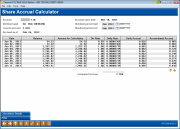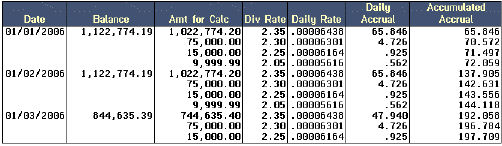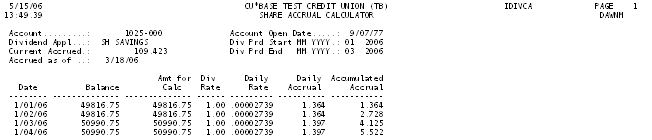# Share Dividend Accrual Calculator

 Screen ID:  Screen Title:  Panel Number: Report Name:IDIVCA-01 Share Accrual Calculator 180 IDIVCAClick here to magnify

This screen appears when Dividend Calculator (F11) is used on the Member Account Inquiry screen for a share or share draft account.

The Share Accrual Calculator displays a “what if” calculation showing how each day's accrual is calculated using the current configuration parameters. It is NOT a historical representation of what actually happened with daily accruals for any given period, but rather serves as a “check and balance” for keeping an eye on how daily dividend amounts are calculated.

The top portion of the screen shows current information about the account, and allows you to enter a dividend period to be verified. The bottom portion of the screen is the calculated sample, showing the daily accrual amount for each day in the period, with a cumulative total for the entire period. The calculator uses transaction history to determine the member's balance as of the time accruals were calculated on each day in the period.

Assuming you are checking calculations for the current dividend period, the Current Accrued and Computed Accrual amounts should match. If they do not, further investigation may be needed.

• You may enter any dividend period (month, quarter, etc.) within the past 12 months. Keep in mind, however, that the calculator will use only the current rate when calculating accruals. If the dividend rate for this product was changed at some point during the past 12 months, or during the dividend period, this calculator will not take that rate change into account.

• NOTE:  This tool will work ONLY for products that use one of the daily accrual dividend calculation methods (simple daily or compound daily).  If this product uses the average daily balance method (use the Calculation Details button to see the Calculation type), no calculation will be performed here.  Learn more about available dividend calculation methods.

## Special Note Regarding Plateau Rate Products

If the product you are viewing is a split-rate plateau product (meaning a blended rate for different portions of the balance), the calculation will be shown differently to detail how much of the balance was calculated at each individual rate.## Field Descriptions

 Field Name Description Current accrued This shows the actual amount currently accrued on this member account. Accrued as of This shows the last date on which accruals were calculated. The Calculator will show sample accrual amounts up to and including this date (if viewing the current dividend period). HINT: Use Calculation Details (F1) to verify whether accruals are calculated during beginning-of-day or end-of-day processing for your credit union. Account open date The date this member account was opened. The Balance column will show 0.00 for each date prior to this open date. Dividend period start Dividend period end Use these field to enter the dividend calculation period you wish to verify. This defaults to the current month and year (MM YYYY) and can be changed to any period (month, quarter, etc.) within the past year. Remember that calculations can only be performed if transaction history exists for that period, so you may not be able to go back a full year if your credit union purges transaction history more frequently. Date This column shows each individual date for which accruals are being calculated. Balance This column shows the actual member balance for each day in the period, determined using transaction history for this member account. Amount for Calculation This column shows the amount that was used in the accrual calculation. This will be the same as the Balance column except for plateau split rates. Click for more information on the display of plateau products. Div Rate This column shows the rate that was used to calculate each sample daily accrual. Daily Rate This column shows the daily rate used in the sample accrual calculation. This is determined by dividing the Div Rate by the number of days in the year (365 or 366). Daily Accrual This column shows the calculated daily accrual amount for each day in the selected dividend period. Remember that this is a calculated rate, not actual history from this member's account. Accumulated Accrual This column shows a cumulative accrual amount for each day in the selected period, calculated by adding the Daily Accrual amount, one day at a time. Computed accrual This grand total adds all of the amounts shown in the Daily Accrual column for the selected period.

## Buttons

 Button Description This key should be used to review the parameters used by CU*BASE when calculating accruals for this account type. Print (F6) This key can be used to print a report showing all accrual calculations for the selected periods. The multiple-page report will be sent directly to your default printer when the key is pressed.

## Report Sample

This report will be automatically sent to your default printer when Print (F6) is used (the report will begin printing after the Calculator screen has been exited).